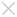English

# Happy CADing with ZW3D: Design a Popular Penguin Doll

August 30, 2016     Happy CADing with ZW3D Penguin Doll

QQ is the name of communication software which is very popular in many countries. Its logo is a little penguin with a red scarf.

Today, I will show you how to design this cute QQ doll with ZW3D software.Figure 1: QQ Doll

Part 1-- Body

Firstly, create  sketch1 on XZ plane, as shown in the left image of figure 2.

Then create the body with Revolve feature, as shown in the right image of figure2.Figure 2: Create the Body

Part2-- Mouth

(1) Create three different datum, as shown in the below figure.Figure 3: Three Different Datum

(2) Create an ellipse in datum1 and datum2 separately. See  figure 4.Figure 4: Ellipse Sketches

(3) Select  datum3 to create  sketch4.

Firstly, click “Reference” feature, select “Curve Intersection”, then pick sketch2 and sketch3 to get  reference points.

Secondly, create a single point, as shown in  figure5.Figure5: Reference Points

At last, apply “Point Curve” feature to create a curve with these five points.Figure 6: Points Curve-Sketch4

(4) Select the YZ datum to create sketch5.

Firstly, apply “Reference” command to create  curve intersection points by selecting sketch2, sketch3 and sketch4. Then create a point curve with these reference points.Figure7: Sketch5

(5) Use “UV Curve Mesh” command to build the surface with these sketch curves. Please make sure the direction of curves should be the same.Figure 8: UV Curve Mesh- Mouth

(6) Show all geometry to get the below result.Figure9: QQ-1

Part 3 Wing

(1) Create  sketch6 on XZ datum. Draw three points, and then create a curve by “Point Curve” feature.

(2) Create  datum4 on the start point of  sketch6, see the below image.

(3) Select  datum4 to insert  sketch7. Draw an ellipse (width10mm, height 12mm).Figure 10: Sketches for Wing

(4) Create the shape with “Sweep” feature. Sketch7 as the profile; Sketch6 as the path curve.Figure11： Sweep

(5) Create a Dome feature at the end of sweep geometry.Figure 12: Dome

(6) Combine the sweep feature and dome feature together.

(7) Mirror the wing to get this result, see  figure 13.Figure 13: QQ-2

Part 4 -- Feet

(1)  Create two new datum by offsetting the XY plane. Offset values are -46mm and -53mm.

(2)  Draw  sketch8 on datum5, which is a half of the ellipse, as shown in figure 14.

(3)  Then do the revolve operation to get a shape.Figure 14: Base Shape of Foot

(4) Draw  sketch9 on datum5, and then extrude it to cut the revolved shape.Figure 15: Extrude Cut

(5) Create  sketch10 on datum5, as shown in the left image of figure 16.

(6) Extract the curves from the edge, as shown in the right image of figure 16.Figure 16: Curves

(7) Create the surface with “UV Curve Mesh”, refer to  figure 17.Figure 17: UV Curve Mesh Surface

(8) Combine the solid shape and this surface together. Then use  datum6 to trim the shape.

(9) Mirror the foot. The result is like  figure 18.Figure 18: QQ-3

Part 5Others

(1)  Create  Sketch 11 on YZ plane, and then project the curve to face, as shown in figure 19.Figure 19: Project Curve to Face

(2)  Create  sketch12 on XZ plane, and then project it to the face, see  figure 20.Figure 20: Project Curve to Face-2

(3) Use the projected curves to split these surfaces.

(4) Create  sketch 13 on XZ plane, and then revolve it with Z axis.Figure 21: Revolve the Sketch

(5)  Change some face attributes, refer to the below image.

(6)  Combine all geometry together, and then add the fillet.Figure 22: Fillet

(7)  Modify some face attributes to get a better look.Figure 23: Final Shape

QQ doll looks like a complex design. In this paper, you will find it can be done easily in ZW3D.

With unique solid-surface hybrid modeling technology, solid geometry and surface geometry can be combined together. Through this case, we can learn the modeling approach of point-curve-surface. Many basic commands are involved in QQ design, such as Sketch, Reference, Revolve, Trim, Project, UV curve mesh and Mirror.

Do you like it? Please try ZW3D to design QQ doll. You will find more fun.